# Behaviour of Gas Molecules

The behaviour of gas molecules is dependent on the properties and laws obeyed by the molecules of the gas. The distribution of molecules in a gas is very different from the distribution of molecules in liquids and solids. There are five properties and five gas laws that govern the behaviour of gas molecules.

## What is Gas?

Gas is defined as a homogeneous fluid which has low density and low viscosity and the volume of the gas is assumed to have the volume equal to the volume of the vessel. The classification of gases are:

• Ideal gas
• Non-ideal gas or real gas

Following are the properties of gases:

 Property Symbol Common units Density d g.l-1 Temperature T K Pressure P mm Hg Volume V cm Amount of gas n mol

Below is the diagram explaining the distribution of molecules in all the three different states of matter.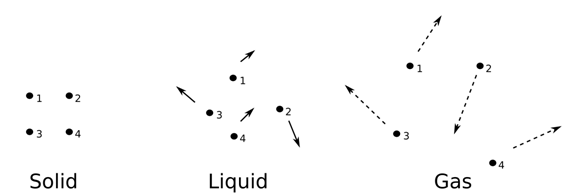## What is Kinetic Theory of Gases?

The behaviour of gas molecules is explained with the help of the kinetic theory of gases. It is the study of gas molecules at the macroscopic level. Following are the five postulated of the kinetic theory of gases:

1. Gas is a composition of a large number of molecules that are constantly in a random movement.
2. The volume of the molecules is negligible as the distance between the gas molecules is greater than the size of the molecules.
3. The intermolecular interactions are also negligible.
4. The collision of molecules with each other and with the walls of the container is always elastic.
5. The average kinetic energy of all the molecules is dependent on the temperature.

Related articles:

## What are the Factors that Affect the Behavior of Gases?

Following are the factors that affect the behaviour of gases:

• Temperature (T)
• Volume (V)
• Pressure (P)
• Quantity (n)

The above-mentioned factors are inter-related and they are given as follows:

• As the temperature increases, the volume of the gas also increases due to an expansion of gas molecules.
• As the temperature decreases, the volume of the gas also decreases due to the contraction of gas molecules.
• As the temperature increases, the pressure of the gas also increases due to an expansion of gas molecules.
• As the temperature decreases, the pressure of the gas also decreases due to the contraction of gas molecules.
• For converting gas either into solid or liquid, the temperature of the gas must be very low or the pressure of the gas must be very high.
• As the quantity decreases, the pressure will also decrease and with an increase in the quantity, the pressure also increases.
• For pressure to decrease, the volume and quantity of the gas should be less.
• For pressure to increase, the volume and quantity of the gas should be more.

## What are Gas Laws?

There are five gas laws that affect the behaviour of gas molecules and they are as follows:

• Boyle’s law
• Charles’s law
• Gay-Lussac’s law
• Ideal gas law

### Boyle’s Law

According to Boyle’s law, the volume of the gas is inversely related to pressure when the amount of gas is fixed at a constant temperature. Mathematical representation of the law is given in the table:

 $$P\propto \frac{1}{V}$$

PV = constant

P1V1 = P2V2 = P3V3 = constant

Where,

• P is the pressure of a gas
• V is the volume of gas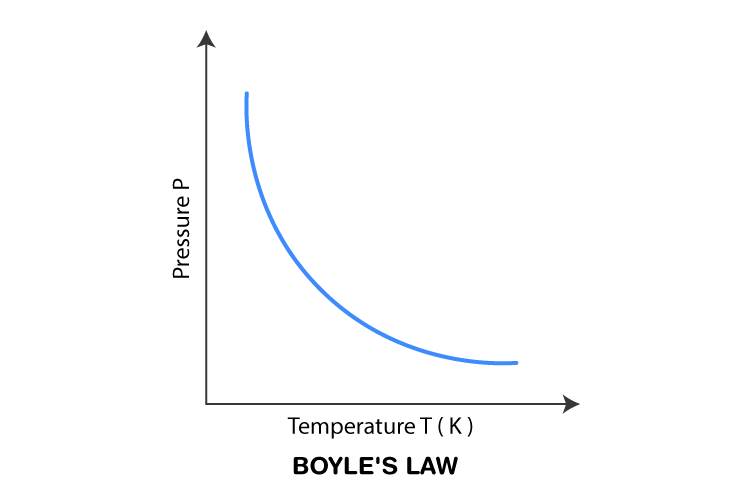### Charles’s Law

According to Charles’s law, the volume of the gas with a fixed mass is directly proportional to the temperature. Mathematical representation of the law is given in the table:

 V ∝ T

Where,

• T is the temperature of a gas
• V is the volume of gas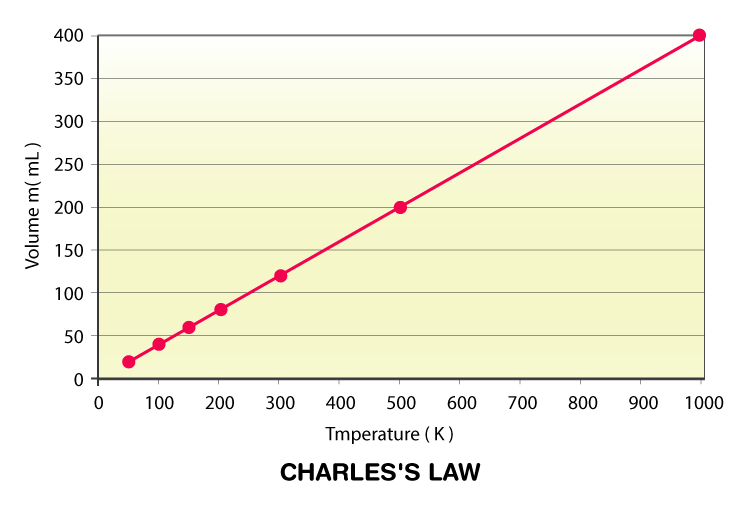### Gay-Lussac’s Law

According to Gay-Lussac’s law, when the volume of the gas is constant, the pressure of a given mass of gas varies directly with the absolute temperature of the gas. Mathematical representation of the law is given in the table:

 $$\frac{P_{1}}{T_{1}}=\frac{P_{2}}{T_{2}}$$

Where,

• T1 is the initial temperature
• P1 is the initial pressure
• T2 is the final temperature
• P2 is the final pressure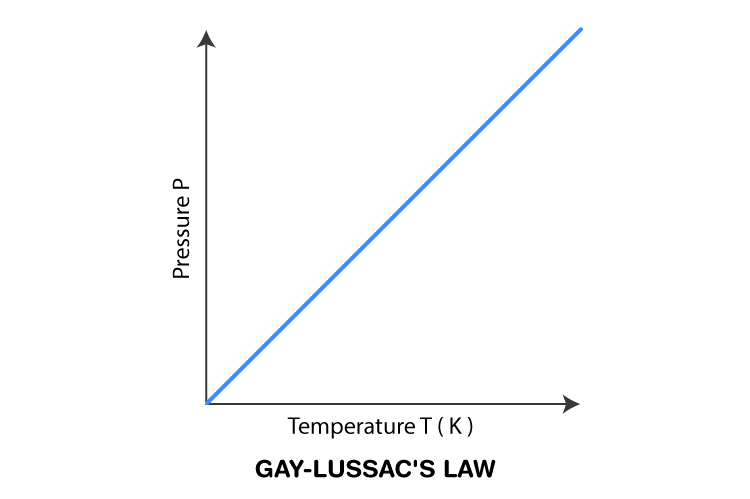According to Avogadro’s law, when the pressure and temperature of the given gas are constant, then the number of moles and the volume of the gas are in a direct relationship. Mathematical representation of the law is given in the table:

 V ∝ n or $$\frac{V}{n}=k$$

Where,

• V is the volume of the gas
• n is the number of moles
• k is the proportionality constant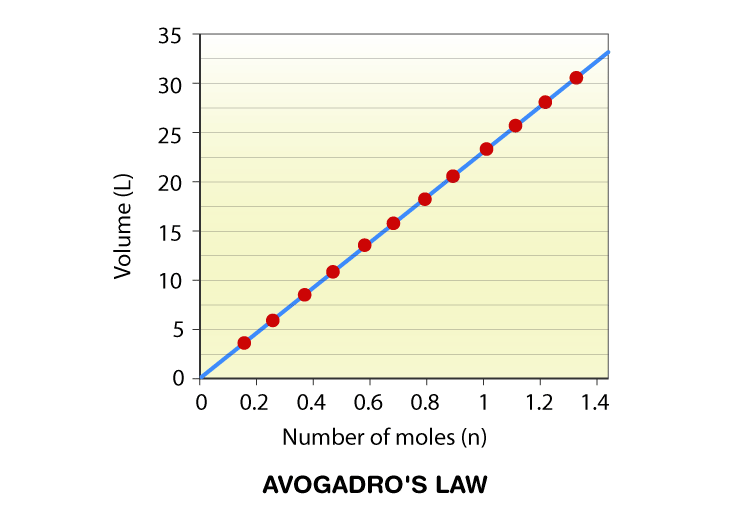### Ideal Gas Law

According to ideal gas law, the product of pressure and volume of one gram molecule of an ideal gas is equal to the product of a number of moles of the gas, universal gas constant and the absolute temperature. Mathematical representation of the law is given in the table:

 PV = nRT = NkT

Where,

• P is the pressure of the gas
• V is the volume of the gas
• n is the number of moles
• R is the universal gas constant = 8.3145 J.mol-1.K-1
• T is the temperature of the gas
• N is Avogadro’s number, NA = 6.0221×1023